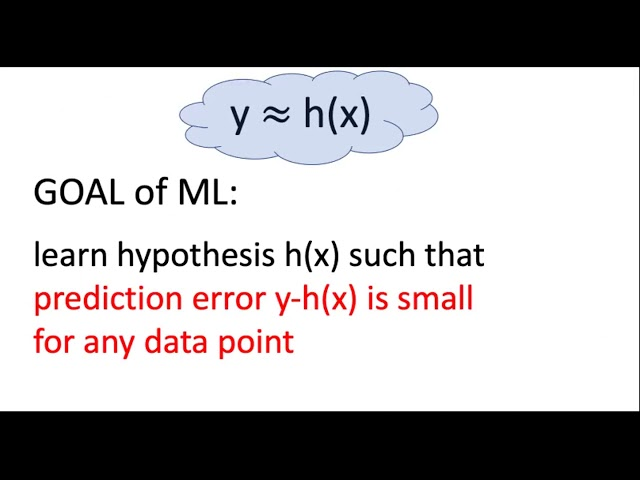# What is Empirical Risk Minimization

##### Understanding Empirical Risk Minimization in AI

Empirical risk minimization or ERM is a popular concept in the field of artificial intelligence and machine learning. It is a statistical learning algorithm used to find the optimal solution out of a set of possible solutions based on sample data. In simple terms, ERM aims at reducing the difference between the actual and the predicted values of a dataset by minimizing the empirical risk.

To understand ERM better, let us look at the basic principles behind it. Empirical refers to something that is based on practical evidence or observation, and risk refers to the possibility of an undesirable outcome. In the context of ERM, empirical risk refers to the probability of error or loss that occurs when we use a particular model to make predictions.

ERM is a widely used concept in the field of AI and machine learning, where the goal is to create an algorithm that can learn from data and make accurate predictions. The basic idea behind ERM is to find a function that can best predict the outcomes of a dataset based on a set of given parameters.

##### The Basic Steps Involved in Empirical Risk Minimization

The ERM process involves a few basic steps that help in identifying the optimal solution for a dataset. These include:

• Identifying the Objective Function: The first step in ERM is to identify the objective function that needs to be optimized. This function is usually a mathematical expression that represents the expected output of a given input.
• Selecting the Model: Once the objective function is identified, the next step is to select the appropriate model that can best predict the outcomes of the dataset. The model is usually a function that predicts an output value based on a given input.
• Determining the Loss Function: A loss function is a measure of the error or deviation between the predicted and actual output values of the dataset. The loss function helps in determining the extent of the error or deviation and plays a crucial role in minimizing the empirical risk.
• Optimizing the Parameters: After the loss function is determined, the next step is to optimize the parameters of the model that affect the output values. The optimization process involves adjusting the parameters to minimize the loss function and improve the accuracy of the model.
##### The Role of Empirical Risk in ERM

The empirical risk in ERM is an important concept that determines the level of error or deviation between the predicted and actual output values of the dataset. The empirical risk is calculated by analyzing the model's performance on a sample dataset and evaluating the performance of the model based on the accuracy of the predictions.

The goal of ERM is to minimize the empirical risk by optimizing the model's parameters and minimizing the loss function. This helps in improving the accuracy of the model and reducing the level of error or deviation between the predicted and actual output values of the dataset.

##### The Advantages of Empirical Risk Minimization

Empirical risk minimization has several advantages that make it a popular concept in the field of artificial intelligence and machine learning. Some of these advantages include:

• Improved Accuracy: ERM helps in improving the accuracy of the model by minimizing the empirical risk and reducing the level of error or deviation between the predicted and actual output values of the dataset.
• Flexible and Adaptive: ERM is a flexible algorithm that can adapt to changing conditions and datasets, making it ideal for use in dynamic environments.
• Efficient: ERM is an efficient algorithm that can process large datasets quickly and accurately, making it ideal for use in real-time applications.
• Easy Implementation: ERM is a simple algorithm that is easy to implement and requires minimal computational resources, making it ideal for use in small-scale applications.
##### The Limitations of Empirical Risk Minimization

Despite its advantages, ERM has certain limitations that need to be considered before using it. Some of these limitations include:

• Overfitting: ERM can sometimes result in overfitting, a situation where the model becomes too complex and fits the training data too closely, resulting in poor performance on new data.
• Underfitting: ERM can also result in underfitting, a situation where the model is too simple and fails to capture the complexity of the dataset, resulting in poor performance on the training data itself.
• Data Bias: ERM can be affected by data bias, where the training dataset may not be representative of the actual data distribution and may result in poor performance on new data.
##### Conclusion

Empirical risk minimization is a powerful concept in the field of artificial intelligence and machine learning that can help in optimizing models and improving their accuracy. ERM works by minimizing the empirical risk or error between the predicted and actual output values of a dataset. However, ERM has certain limitations like overfitting, underfitting, and data bias that need to be considered before using it.Despite its limitations, ERM remains a popular algorithm due to its flexibility, efficiency, and easy implementation, making it ideal for a wide range of applications in dynamic environments.